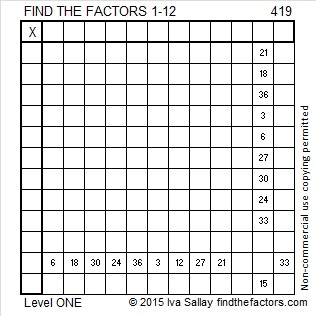# 419 and Level 1

2^419 (mod 419) = 2 so 419 is VERY LIKELY a prime number. Scroll down past the puzzle to know for sure.Print the puzzles or type the factors on this excel file: 12 Factors 2015-03-09

• 419 is a prime number.
• Prime factorization: 419 is prime.
• The exponent of prime number 419 is 1. Adding 1 to that exponent we get (1 + 1) = 2. Therefore 419 has exactly 2 factors.
• Factors of 419: 1, 419
• Factor pairs: 419 = 1 x 419
• 419 has no square factors that allow its square root to be simplified. √419 ≈ 20.4695How do we know that 419 is a prime number? If 419 were not a prime number, then it would be divisible by at least one prime number less than or equal to √419 ≈ 20.4695. Since 419 cannot be divided evenly by 2, 3, 5, 7, 11, 13, 17, or 19, we know that 419 is a prime number.## 4 thoughts on “419 and Level 1”

1.davescarthin

No factors, not a sum of two squares not a Loeschian number (a^2 + ab +b^2) – flinty, indivisible, primly prime 419!

•abyssbrain

Well. at least, (419×2^2n)/3 cannot be prime for any value of n. (I wont post the proof though since its too long 🙂 )

•davescarthin

Crikey! hang on, though, your expression isn’t an integer.

Would you like to specify two colours that go together well for your tessellation, specified either as CMYK or RGB or just as “blobs” I can copy.

•abyssbrain

I think that CMYK would be good.

This site uses Akismet to reduce spam. Learn how your comment data is processed.